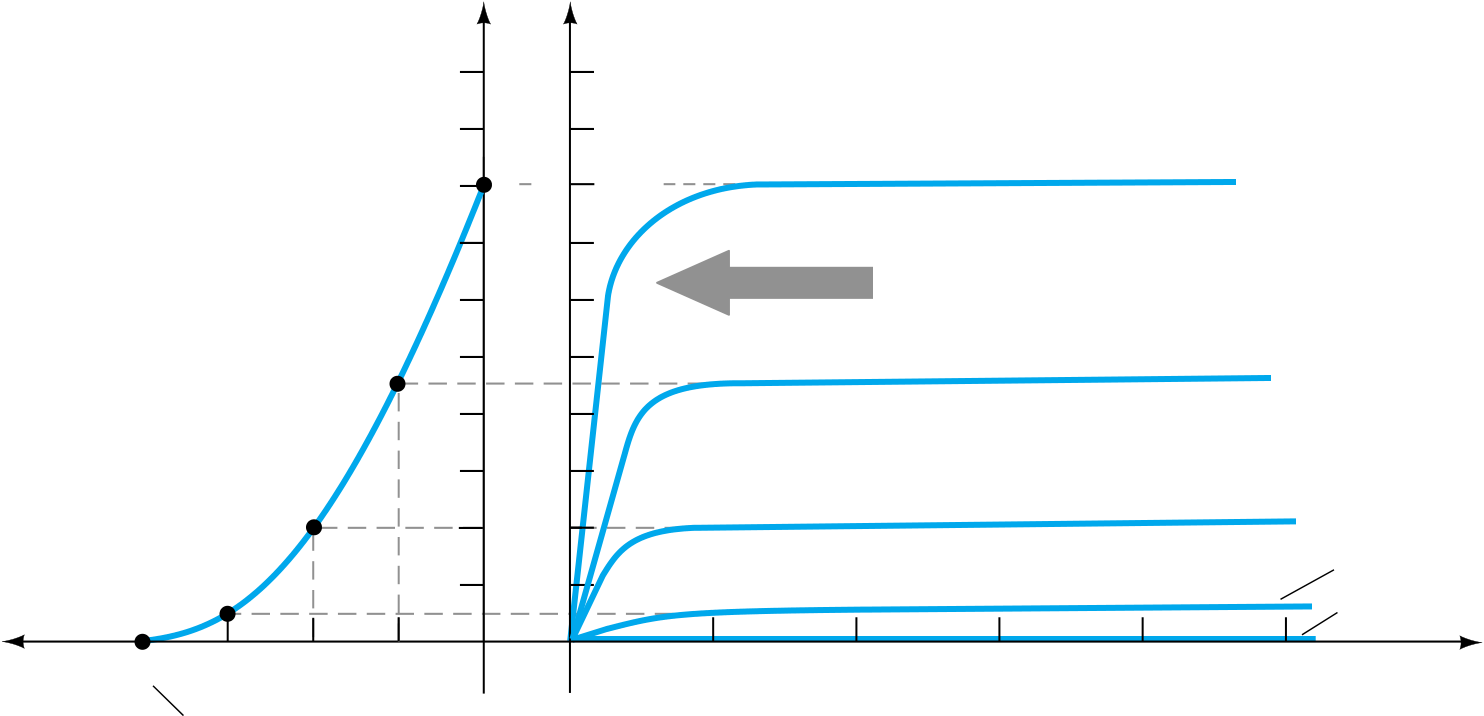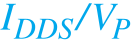Rating : ⭐⭐⭐⭐⭐
Price : \$10.99
Language:EN
Pages: 2

# Equation form variableconstantwilliam bradford shockleyICf(IB) � �IB

constant

(5.2)Unfortunately, this linear relationship does not exist between the output and input

quantities of a JFET. The relationship between ID and VGS is defined by Shockley’s

control variable (5.3)

The squared term of the equation will result in a nonlinear relationship between ID and VGS, producing a curve that grows exponentially with decreasing magnitudes of VGS.

For the dc analysis to be performed in Chapter 6, a graphical rather than mathe-matical approach will in general be more direct and easier to apply. The graphical ap-proach, however, will require a plot of Eq. (5.3) to represent the device and a plot of the network equation relating the same variables. The solution is defined by the point of intersection of the two curves. It is important to keep in mind when applying the graphical approach that the device characteristics will be unaffected by the network in which the device is employed. The network equation may change along with the intersection between the two curves, but the transfer curve defined by Eq. (5.3) is un-affected. In general, therefore:

ID (mA)
10 10
9

9

IDSS 8 8

IDSS

7
6
5

VGS = –1 V

4

4

Obtaining the
3
2
1

1

VGS (V)

– 4

–3 –2 –1 0
5 10 15 20
VDS
ID = 0 mA, VGS

= VP

5.3

Transfer Characteristics

When VGSVP � �4 V, the drain current is zero milliamperes, defining another point on the transfer curve. That is:

When VGSVP, ID0 mA.

220 and Eq. (5.3):

2 IDIDSS�1 � �V V�� G

(5.4)

Field-Effect Transistors

IDIDSS | VGS � 0 V
Chapter 5
How It Works